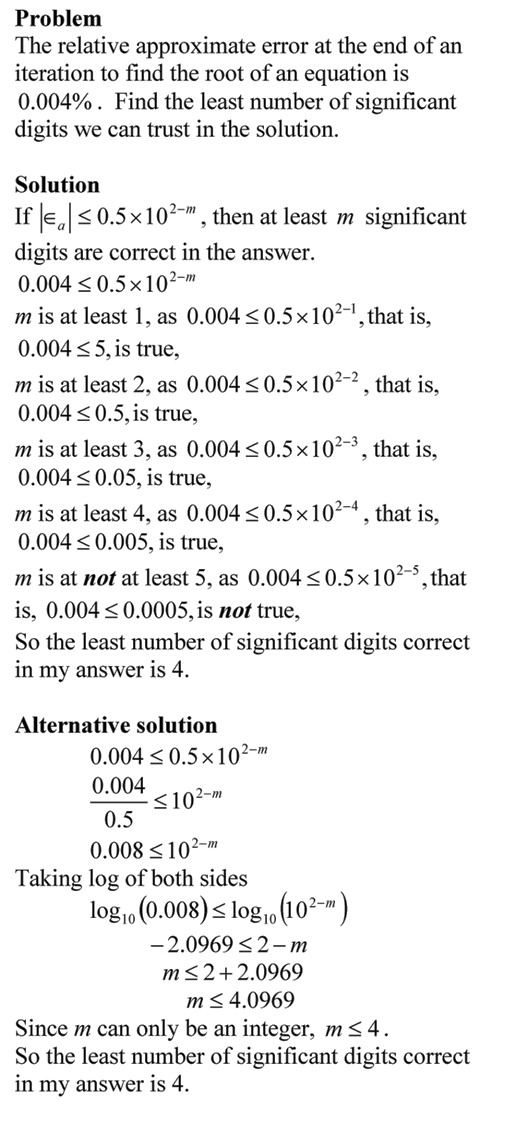# Example: How many significant digits are correct in my answer?

In the previous post I showed you the theorem that tells us how many significant digits are correct in our numerical solution that is iterative in nature.  In this post, we illustrate this with an example.__________________________________________
This post is brought to you by Holistic Numerical Methods: Numerical Methods for the STEM undergraduate at http://nm.mathforcollege.com, the textbook on Numerical Methods with Applications available from the lulu storefront, the textbook on Introduction to Programming Concepts Using MATLAB, and the YouTube video lectures available at http://nm.mathforcollege.com/videos.  Subscribe to the blog via a reader or email to stay updated with this blog. Let the information follow you.

Posted on Categories Numerical Methods

## 0 thoughts on “Example: How many significant digits are correct in my answer?”

1.Lebann says:

Hi! what is the name of theorem? The theorem name will be helpful but if you could also provide a derivation or a reference which derives this it would be even better, Thanks in advance!

2.Lebann says:

Hi! what is the name of theorem? The theorem name will be helpful but if you could also provide a derivation or a reference which derives this it would be even better, Thanks in advance!